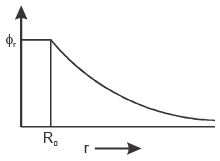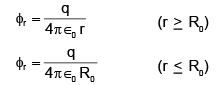# The electrostatic potential (φr) of a spherical symmetric system, kept at origin, is shown in the adjacent figure, and given asW hich of the following option(s) is/are correct?a)For spherical region r < R0, total electrostatic energy stored is zero.b)W ithin r = 2R0, total charge is q.c)There will be no charge anywhere except at r = R0.d)Electric field is discontinuous at r = R0.Correct answer is option 'A,B,C,D'. Can you explain this answer? Related Test: Coded Inequalities MCQ - 1

## IIT JAM QuestionSaumodip Den Dec 13, 2020
1_ in the sphere the energy of the electrostatic will be cancelled in each other. 2 the outer orbit the charge will be q there are no changes.3 due to induction of charge